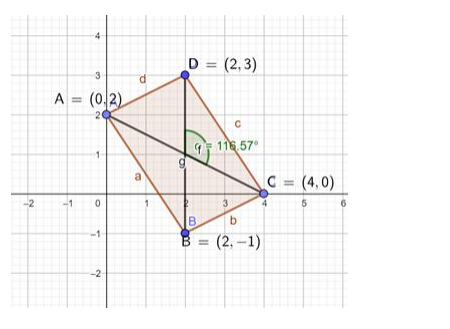# If θ is the angle between the diagonals of a parallelogram ABCDQuestion:

If θ is the angle between the diagonals of a parallelogram ABCD whose vertices are A(0, 2), B(2,-1), C(4,

Solution:

Given points of the parallelogram are A(0, 2), B(2,-1), C(4, 0) and D(2, 3)

slope $=\left(\frac{\mathrm{y}_{2}-\mathrm{y}_{1}}{\mathrm{x}_{2}-\mathrm{x}_{1}}\right)$The slope of diagonal $A C=\left(\frac{0-2}{4-0}\right)=\frac{-2}{4}=\frac{-1}{2}=m_{1}$

The slope of diagonal $B D=\left(\frac{3+1}{2-2}\right)=\frac{4}{0}=\infty=m_{2}$

So diagonal BD is perpendicular to X-axis. Hence it is parallel to Y-axis.

Product of slope of two diagonals is equal to $-1$.

$\mathrm{m}_{1} \times \mathrm{m}_{2}=-1$

$\Rightarrow\left(\frac{-1}{2}\right) \times \tan \theta=-1$

$\Rightarrow \tan \theta=2$

Hence proved.